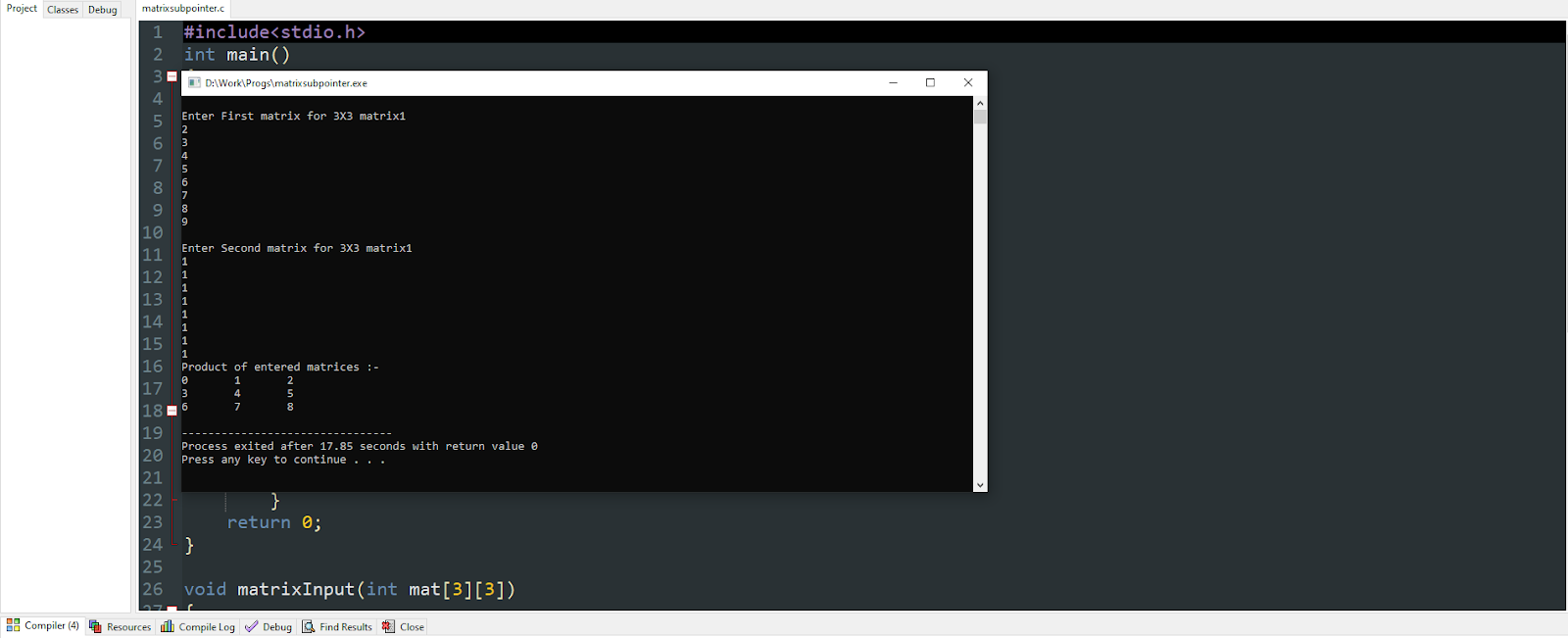# How to subtract two matrices using a pointer?

Here we are going to solve substract two matrices using a pointer. How to solve Let’s begin-

## What is a matrix?

A matrix is a 2D array which is used to store and display data in a grid format. For example:

The above illustrates a matrix where a number for example 10 is denoted by arr, the first one denotes the row number and the second one denotes the column number.

### What is a matrix subtraction?

When two matrices are subtracted of the same type and stored in a resultant matrix it is known as matrix subtraction. Two matrices can be subtracted if they have the same dimension. In the program we will follow the technique shown below:

``res=arr-arr1;``

It is illustrated as the number in the first row and the first column of the first matrix will be subtracted to the number in the first row and first column of the second matrix and will be stored in the resultant matrix.

### Explanation:

• We take three matrices int a as the first matrix int b as the second matrix and the resultant matrix as c.
• We take loop variables as i and j, to print the resultant matrix.
• We take the input of the two matrices in matrixInput function, where they are stored and their addresses are stored in the pointer variable. This operation is direct performed in the scanf(“%d”, (*(mat + row) + col)) function.
• Then the three matrices are sent to the matrixsubtract function (the third matrix is sent to store the result).
• The subtraction operation takes place with the help of the pointers illustrated as *(*(res + i) + j) =(*(*(mat1 + i) + j))-(*(*(mat2 + i) + j)), this states that each variable of both the matrices are subtracted with the help of their memory address and the subtracted value is generated whose address is stored in the resultant matrix.
• In the end, we print the resultant matrix stored in c, with the help of addresses i.e., the pointer.
• Hence we complete the program of matrix subtraction with a pointer.
```#include<stdio.h>
int main()
{
int a,b,c;
int i,j;
printf("\nEnter First matrix for 3X3 matrix");
matrixInput(a);

printf("\nEnter Second matrix for 3X3 matrix");
matrixInput(b);

matrixsubtract(a,b,c);

printf("Product of entered matrices :-\n");

for(i=0;i<3;i++)
{
for(j=0;j<3;j++)
printf("%d\t",*(*(c + i) + j));
printf("\n");
}
return 0;
}

void matrixInput(int mat)
{
int row, col;
for(row=0;row<3;row++)
{
for(col=0;col<3;col++)
{
scanf("%d", (*(mat + row) + col));
}
}
}

void matrixsubtract(int mat1, int mat2, int res)
{
int i,j;
for(i=0;i<3;i++)
{
for(j=0;j<3;j++)
{
*(*(res + i) + j) =(*(*(mat1 + i) + j))-(*(*(mat2 + i) + j));
}
}
}```

Here in the above program, we have taken a 3X3 matrix to show the example of matrix subtraction.

The function matrixInput takes the input of the matrix one by one using the loop when row=0 and column=0 it takes the input of the 1st variable of the row and then the value of column is incremented where row=0 and column=1 taking the input of the 2nd variable of the row and then at last row=0 column=2 taking the input of the 3rd variable of the row.

The row gets incremented now where row=1 and column=0, it takes the input of the 1st variable of the row and then the value of column is incremented where row=1 and column=1 taking the input of the 2nd variable of the row and then at last row=1 column=2 taking the input of the 3rd variable of the row.

Then the row gets incremented now where row=2 and column=0, it takes the input of the 1st variable of the row and then the value of column is incremented where row=2 and column=1 taking the input of the 2nd variable of the row and then at last row=2 column=2 taking the input of the 3rd variable of the row.

The whole input operation uses a pointer where the address of the values are stored in the pointer variable.

Then the values are sent to the matrixsubtract function and the subtraction operation is carried out, where the values of each row and column are subtracted to the same row and column of the second matrix such as: res=mat1-mat2 and so on. This subtraction operation is carried out by the help of pointer where the subtraction takes place and the address is stored of the value i.e., *(*(res + i) + j) =(*(*(mat1 + i) + j))-(*(*(mat2 + i) + j)) and the resultant address of the subtracted variables are stored in the res matrix.

Hence this is how the subtraction takes place. Later in the main function, the resultant matrix c is printed to get the output of the addition.

Output:This is how matrix subtraction is done through a pointer. There may also be some other procedure depending on the logic part of the user.##### Soham Malakar

Hello my name is Soham Malakar am currently pursuing BCA

Coding is one of my favorite hobbies.
I love to code and an enthusiast to learn different types of programming languages.
I love to get intoxicated in the world of technology and video games.
A quick learner possessing better results.

Articles: 40